# How to determine power gain and voltage gain in RF systems

Other Parts Discussed in Post: LMH6521, LMH6515

I’m hearing more and more customers ask ”how does gain through a signal chain change with different load impedances?"  And, "when does voltage gain and power gain coincide when measured in dB?” I wanted to share the answers with the Analog Wire audience in case any of you have the same quesitons. So, here we go…..

In a single-ended signal path with 50 Ohms termination, the gain calculations are very easy because the voltage gain (20* log (Vout/Vin)) is equal to the power gain (10* log (Pout/Pin)).  Things become a bit more complicated though when the impedance of the load, or source, changes.   For example, in many radio receiver channels the 50 Ohm single-ended signal is converted to a 200 Ohm differential signal before it it digitized with a high performance ADC, such as the ADC16DV160.

In addition, there are two main types of amplifiers, voltage output amplifiers like the LMH6521, and current output amplifiers like the LMH6515.   The calculations below show how these two different kinds of amplifiers react to different load conditions.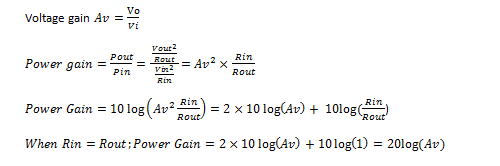Voltage output amplifiers are the most common amplifiers in non-RF systems and have been immortalized by the classic operational amplifier (op amp). Note that both current feedback and voltage feedback op amps have a voltage output architecture.   With recent developments in bipolar transistor technology, op amps and their derivatives are useful up to 2 GHz or higher. As a result,  they are finding their way into RF and IF signal paths.  Because an op amp has infinite input impedance and zero output impedance,  the power gain of an op amp is not normally specified, rather the gain is given as a voltage gain (Av).  A common gain setting is 6dB, where the output voltage is 2x the input voltage.  Note that this gain does not specify an input load condition or an output load condition.  Because a voltage alone is not sufficient to calculate power, power gain can not be calculated using only a voltage gain.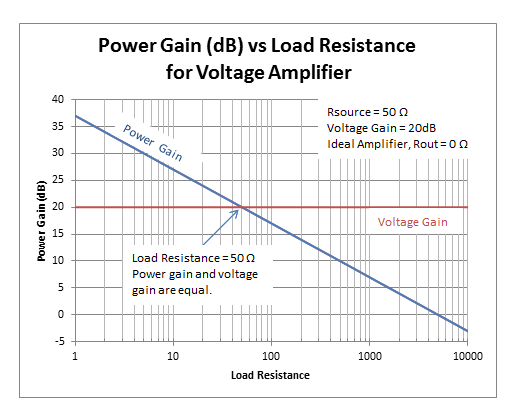Figure 1 ideal voltage amplifier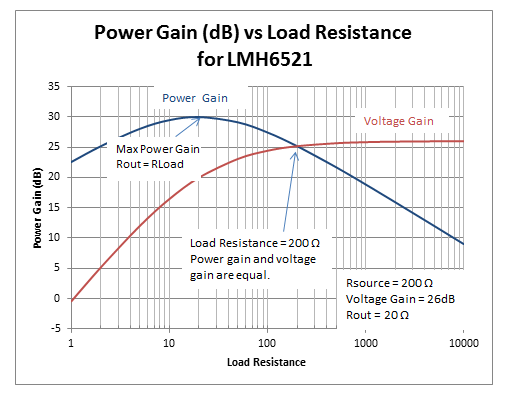Figure 2 example voltage amplifier: LMH6521

Current output  amplifiers are another common type of RF amplifier beacause a given input signal a given output current is produced.  There are two common configurations in one configuration Iout = (in * Gain) in the other configuration Iout = (Vin * gain).  The latter is more common and in that case the gain is called transconductance (gm). In transconductance  amplifier calculations, both the voltage gain and power gain are dependent on load conditions. (Example Amplifier LMH6515 Rin = 200 Ω, Rout = 200 Ω or 400 Ω, Maximum  gain = 0.1 A/V)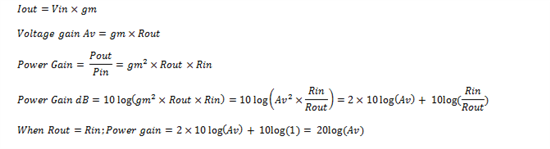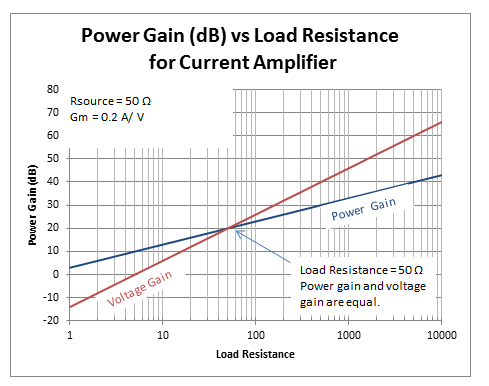Figure 3 ideal current amplifier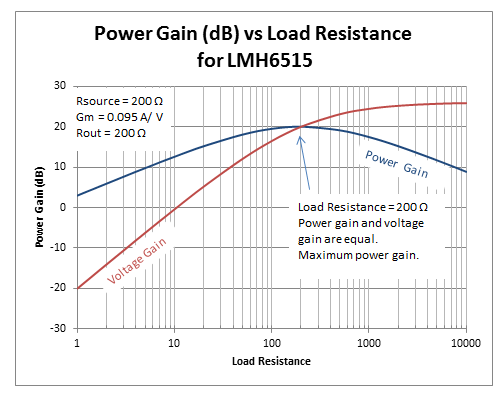Figure 4 example current amplifier: LMH6515

For both amplifier topologies, voltage gain in dB and power gain in dB are only equal when the input and output impedances are the same.   With current amplifiers, however, both voltage gain and power gain will change with load conditions, while with voltage amplifiers only power gain changes with load.

As an exercise for the reader, prove that a back terminated load cuts both voltage gain and power gain by 6dB and let me know how it goes!

• Hi Loren,

Sorry for the super late reply (I didnt get a notification that u actually answered my question).

This is coming from a hobbiest. What is the mathematical relationship between power gain and Load resistance?Also what is the mathematical relationship between voltage gain and load resistance? I see u have plotted it in 4 graphs. But in yur equations I dont see Rload, just Rout. Im assuming they are not the same. )I ma aware this might be a noob question)

Cheers,

Stevan

• Hello William,

I suppose now is a good time to review the exercise question.  Using a back terminated load is tricky because when you take a first look at the circuit it appears that the voltage loss is 6dB and the power loss is only 3dB.  The power loss is tricky because even though the matching resistor only absorbs 1/2 of the total delivered power that is not the whole story.

Let's look at the example of a video cable.  Because of transmission line effects it is best to provide double termination of the cable.  However,  If you could connect the signal source to the 75 Ohm load with a 1Vpp signal the output power goes entirely into the load.

When you add termination resistors to match the cable you lose 1/2 of your power into the matching resistor and you also lose 1/2 of your voltage, so that your 75 Ohm load now only gets 0.5Vpp.    You could have had 1Vpp into a 75 Ohm load, but now you only get 0.5Vpp into your 75 Ohm load.

If you look at a power chart a 1Vpp signal into 75 Ohms is 2.2dBm, while a 0.5Vpp signal into 75 Ohms is -3.8dB (2.22dBm - -3.80dBm = 6.02dB)  Your termination resistors have cost you 6dB of "possible" power, even though they are only absorbing 3dB of delivered power.

The difference between "possible" and delivered is because in the back terminated example the 1Vpp signal is no longer driving a 75 Ohm load, but rather is driving two 75 Ohm loads in series.  Each load gets 1/2 of the delivered power, but the possible power was also decreased by 1/2.

Naturally, you will increase the output voltage of your amplifier to make up for the lost voltage in the termination resistor.  So in this case you would now be driving a 2Vpp signal into your video cable.  Even though the termination resistors only consume 3dB of the output power you needed a 6dB increase in output voltage to keep your load power constant.  (2Vpp into 150 Ohms = 5.23dBm while  1Vpp into 75 Ohms = 2.22dBm)

• Hello Stevan,

For the purposes of this blog I did not consider Source resistance.  The graphs that show real devices have the device output resistance included in the calculation.  With a given IC such as the LMH6517 the output resistance is fixed and close to zero and so it does not factor into the calculation very much.  On a part like the LMH6521 the output resistance is fixed, but is not negligible, so it must be considered.  For the LMH6515 the output resistance can be chosen from two different values, so it is good to know the tradeoffs of the selections.

I did not include source resistance calculations because many times test equipment is already calibrated to take into account a matched load.  For example, an RF signal generator wit a 50 Ohm output will deliver the indicated power into a 50 Ohm load.  It is correct that if you connect a different load to the signal generator you will get a different power, so this is an important consideration.

When we make measurements most of the time we take great care to provide matched conditions for our test equipment.   For a 200 Ohm DVGA we would use a 1:4 balun on the input to convert the 50 Ohm signal generator signal to 200 Ohms for the DVGA.  If magnetic components do not work we will use a resistive pad to provide the impedance matching.

• Great article Loren. Thank you.

My question is regarding the Rin and Rsource (Rout and Rload) you use in your calculations and graphs respectively. I think I am correct to assume that Rin and Rsource (Rout and Rload) are not the same values necessarily. What is the influence of these 4 impedances on the power gain and voltage gain (for voltage and current amps) if Rsource is different to Rin and Rout is different to Rload (impedance mismatch condition). What Im really trying to understand here is what equations did you use to plot graphs 1 and 3. For example, you plot Power Gain and voltage gain in Fig. 1 using Av, Rin, Rout, Rsource and Rload but only the power gain equations with Av, Rin and Rout are presented. Same goes for power gain plot in Fig 3 where you use gm,, Rsource, Rout, Rin, Rload.

Thanks.

• Thank you.  This is the kind of  thing that is most helpful.  Its basic, straightforward and well written.  It goes into my notebook.  I hope you tell us  the answer to your exercise.